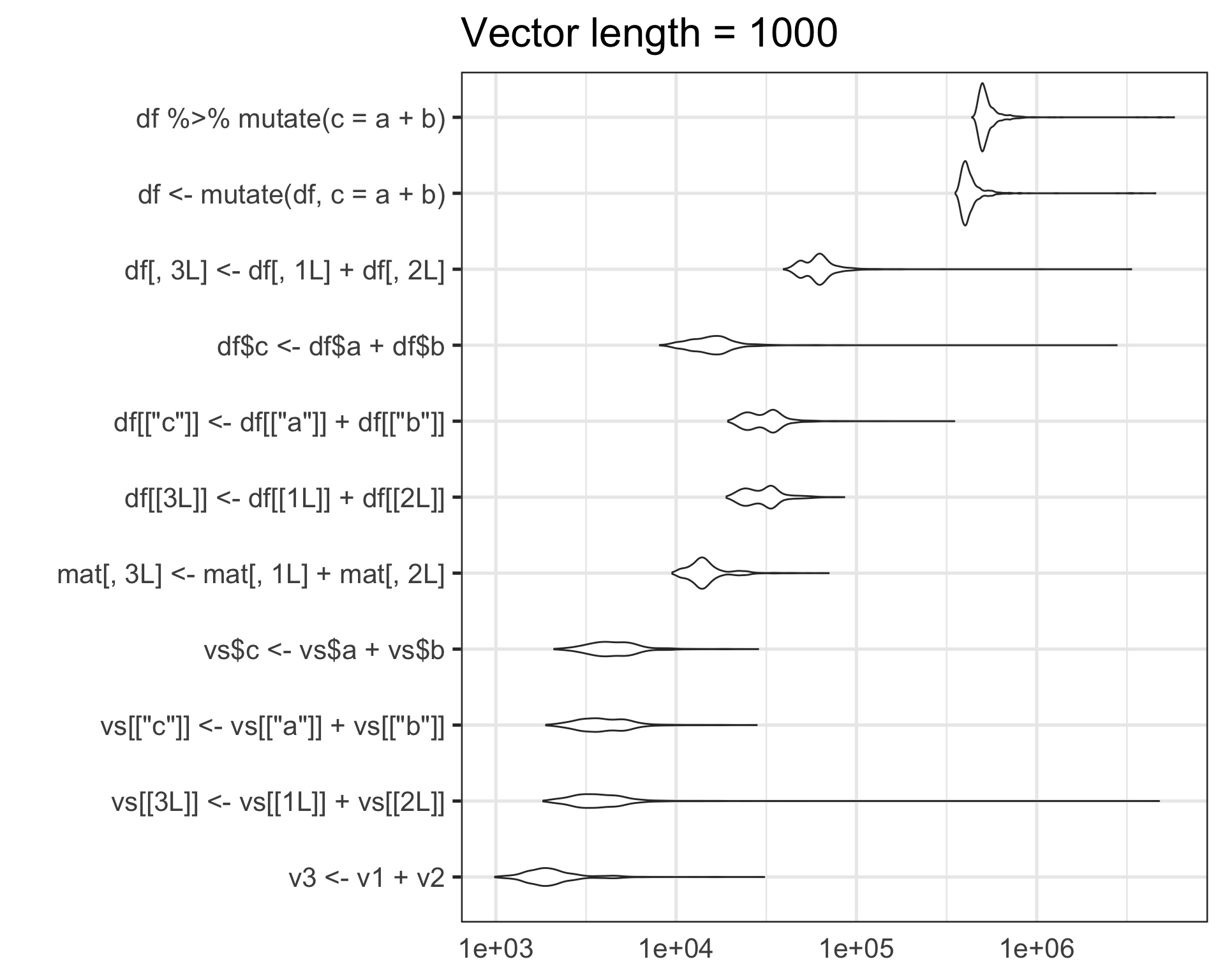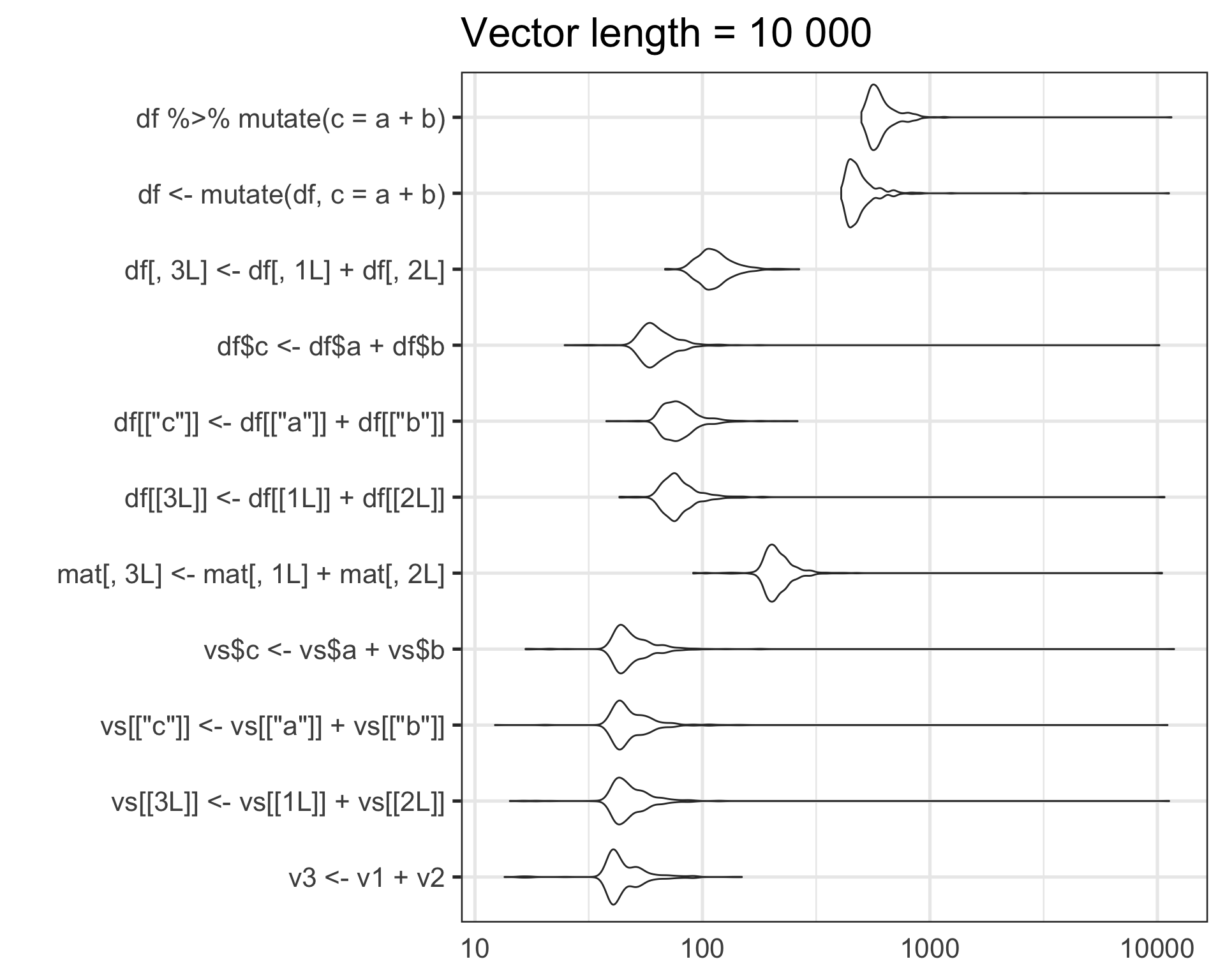# Benchmark mutate - vector, matrix, data.frame

## Introduction

I’m looking at some numeric code that’s going to be in an inner loop and just wanted to get an idea of the relative speed differences of different approaches (Rcpp may be an option in the future, but not right now.)

Benchmark Context:

• The vector lengths are in the range 1000-10000
• This operation will be performed of the order of 1000 times.
• Key question: Is the speed gained by working with raw lists of vectors worth losing the convenience/safety of the data.frame?
• Disclaimer: This is an exploration for my particular use case, on my particular computer and not meant as definitive answer as to which is the fastest approach in all situations.

Data structures I’m considering:

• List of vectors of the same length
• Matrix
• data.frame

While I’m not considering raw/naked vectors (as I need at least some structure to my data structures!), I have included them in the benchmark as a baseline i.e. v3 <- v1 + v2.

## Create test data

• create_test_data() is used to generate data for the benchmark
• I have included an example small dataset in the 4 different formats
#-----------------------------------------------------------------------------
# Create a list of N vectors of length L
#-----------------------------------------------------------------------------
create_test_data <- function(N, L) {
set.seed(1)
seq(N) %>%
purrr::map(~runif(L)) %>%
set_names(letters[1:N])
}

vs  <- create_test_data(3, 5)
mat <- do.call(cbind, vs)
df  <- data.frame(vs)
v1  <- vs[]
v2  <- vs[]

vs
$a  0.2655087 0.3721239 0.5728534 0.9082078 0.2016819$b
 0.89838968 0.94467527 0.66079779 0.62911404 0.06178627

$c  0.2059746 0.1765568 0.6870228 0.3841037 0.7698414 mat  a b c [1,] 0.2655087 0.89838968 0.2059746 [2,] 0.3721239 0.94467527 0.1765568 [3,] 0.5728534 0.66079779 0.6870228 [4,] 0.9082078 0.62911404 0.3841037 [5,] 0.2016819 0.06178627 0.7698414 df  a b c 1 0.2655087 0.89838968 0.2059746 2 0.3721239 0.94467527 0.1765568 3 0.5728534 0.66079779 0.6870228 4 0.9082078 0.62911404 0.3841037 5 0.2016819 0.06178627 0.7698414 ## Benchmark dataset - length = 1000 • dataset: 3 vectors with 1000 elements each res <- microbenchmark( v3 <- v1 + v2, vs[[3L]] <- vs[[1L]] + vs[[2L]], vs[['c']] <- vs[['a']] + vs[['b']], vs$c        <- vs$a + vs$b,
mat[,3L]    <- mat[,1L]    + mat[,2L],
df[[3L]]    <- df[[1L]]    + df[[2L]],
df[['c']]   <- df[['a']]   + df[['b']],
df$c <- df$a        + df\$b,
df[,3L]     <- df[,1L]     + df[,2L],
df          <- mutate(df, c = a + b),
df %>% mutate(c = a + b),
times = 1000
)## Benchmark dataset - length = 10000

• dataset: 3 vectors with 10000 elements each## Results

For this particular use case, on my machine:

• Raw lists of vectors are about an order of magnitude faster than matrix or data.frame operations
• data.frame operations via base R are about an order of magnitude faster than dplyr

For this particular task, I think the order of magnitude speedup of using raw lists is worth the hassle/danger/inconvenience of manually handling vectors in lists.